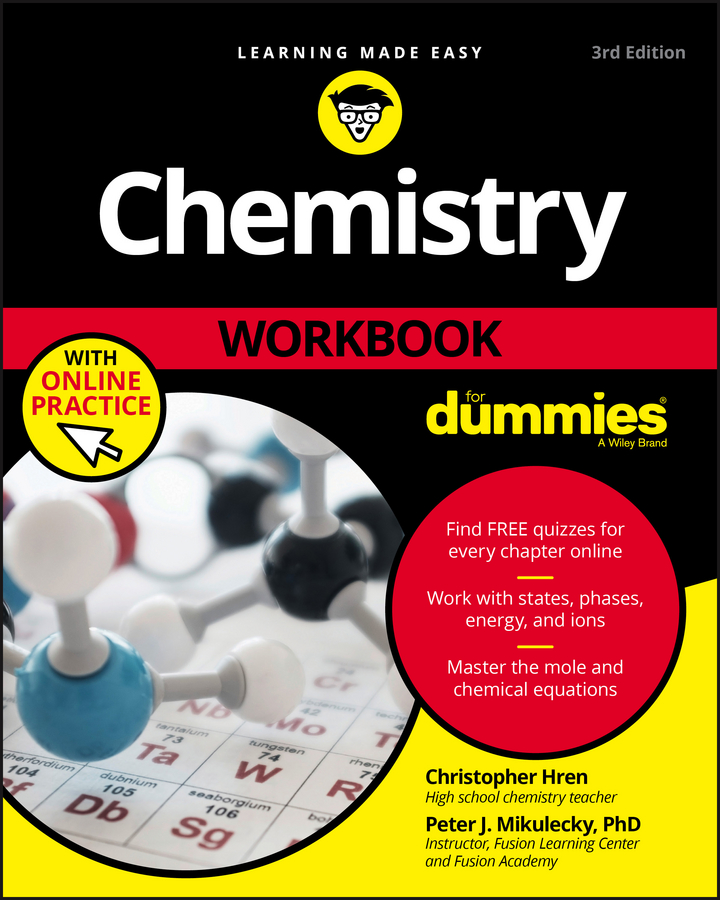# Chemistry Workbook For Dummies with Online Practice

Authors:
Published: April 17, 2017

## Overview

Take the confusion out of chemistry with hundreds of practice problems

Chemistry Workbook For Dummies is your ultimate companion for introductory chemistry at the high school or college level. Packed with hundreds of practice problems, this workbook gives you the practice you need to internalize the essential concepts that form the foundations of chemistry. From matter and molecules to moles and measurements, these problems cover the full spectrum of topics you'll see in class—and each section includes key concept review and full explanations for every problem to quickly get you on the right track. This new third edition includes access to an online test bank, where you'll find bonus chapter quizzes to help you test your understanding and pinpoint areas in need of review. Whether you're preparing for an exam or seeking a start-to-finish study aid, this

workbook is your ticket to acing basic chemistry.

Chemistry problems can look intimidating; it's a whole new language, with different rules, new symbols, and complex concepts. The good news is that practice makes perfect, and this book provides plenty of it—with easy-to-understand coaching every step of the way.

• Delve deep into the parts of the periodic table
• Get comfortable with units, scientific notation, and chemical equations
• Work with states, phases, energy, and charges
• Master nomenclature, acids, bases, titrations, redox reactions, and more

Understanding introductory chemistry is critical for your success in all science classes to follow; keeping up with the material now makes life much easier down the education road. Chemistry Workbook For Dummies gives you the practice you need to succeed!

Chemistry Workbook For Dummies Cheat Sheet

Getting through a chemistry class involves a range of science skills and procedures. You use exponential and scientific notation, analyze atomic structures, name compounds, convert to and from moles, and draw Lewis dot structures. Is there anything you don't do in chemistry?

## Articles From The Book

19 results

Chemistry Articles

## Conversion factor table

The following table includes some useful conversion factors.

## Using conversion factors example

The following example shows how to use a basic conversion factor to fix non-SI units. Dr. Geekmajor absentmindedly measures the mass of a sample to be 0.75 lb and records his measurement in his lab notebook. His astute lab assistant, who wants to save the doctor some embarrassment, knows that there are 2.2 lbs in every kilogram. The assistant quickly converts the doctor’s measurement to SI units. What does she get? The answer is 0.34 kg. Let’s try another example. A chemistry student, daydreaming during lab, suddenly looks down to find that he’s measured the volume of his sample to be 1.5 cubic inches. What does he get when he converts this quantity to cubic centimeters? The answer is 25 cm3. Rookie chemists often mistakenly assume that if there are 2.54 centimeters in every inch, then there are 2.54 cubic centimeters in every cubic inch. No! Although this assumption seems logical at first glance, it leads to catastrophically wrong answers. Remember that cubic units are units of volume and that the formula for volume is Imagine 1 cubic inch as a cube with 1-inch sides. The cube’s volume is Now consider the dimensions of the cube in centimeters: Calculate the volume using these measurements, and you get This volume is much greater than 2.54 cm3! To convert units of area or volume using length measurements, square or cube everything in your conversion factor, not just the units, and everything works out just fine.

Chemistry Articles

### How to Add and Subtract with Exponential Notation

In chemistry, you can add and subtract extreme numbers by using exponential notation, and expressing your numbers as coefficients of identical powers of 10. To wrestle your numbers into this form, you may need to use coefficients less than 1 or greater than 10.

To add two numbers by using exponential notation, you begin by expressing each number as a coefficient and a power of 10. In this example, you add these numbers, by following these steps:
1. Convert both numbers to the same power of 10.
3. Join your new coefficient to the shared power of 10.

## Subtracting with exponential notation

To subtract numbers in exponential notation, you follow the same steps but subtract the coefficients. Here’s an example:

0.0743 – 0.0022

To perform the subtraction, follow these steps:
1. Convert both numbers to the same power of 10.
2. Subtract the coefficients. 7.43 – 0.22 = 7.21
3. Join your new coefficient to the shared power of 10.
Now try a few practice questions.